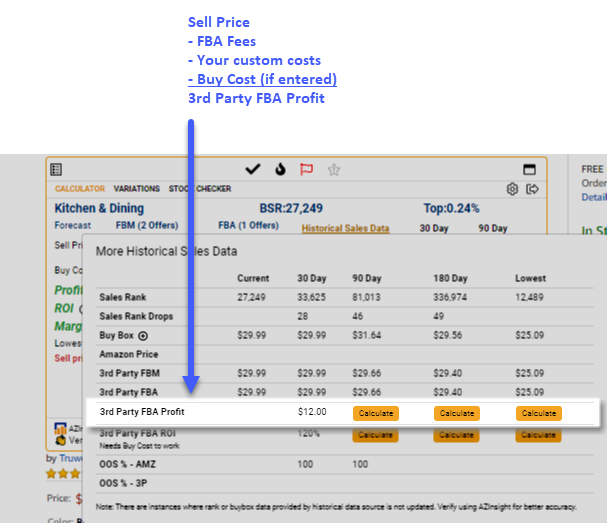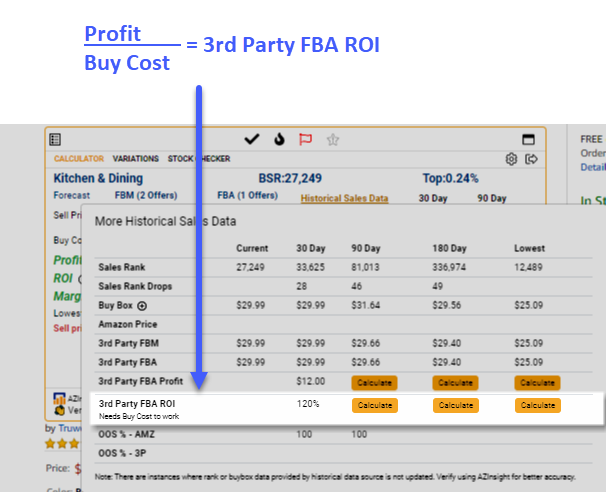# How is the 3rd Party FBA Profit and 3rd Party FBA ROI calculated on the historical data section?

### To calculate the 3rd Party FBA Profit AZInsight uses the formula

Sell Price - FBA Fees - Your custom costs - Buy Cost (if any is entered in the calculator screen)

The Sell Price and Buy Cost used for this calculation come from what is entered on the calculator screen.### To calculate the 3rd Party FBA ROI, AZInsight uses the formula

Profit / Buy Cost (if any is entered in the calculator screen)

Note: If there is no Buy Cost entered on the calculator or it is zero, then AZInsight will not be able to calculate the 3rd Party FBA ROI